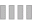A-A+

# 快速輸入特殊文字表情符號

2010年05月01日

STEP1XP系統下將輸入法換成新注音，之後按住鍵盤上TAB鍵上方的~」鍵，接著新注音會出現提示，告訴你多功能前引導字元的用法，由於我們要輸入內碼，所以再按下鍵盤上的「U」鍵。

NOTE：按「U」表示要用Unicode碼來輸入符號，而「B」則可改用一般的big5內碼來輸入符號(如同內碼輸入法)，你可視自己的使用習慣來選擇。

STEP2接著微軟新注音會另外出現一小排灰色輸入區，我們直接按下鍵盤上的數字鍵，比如輸入2605，就會出現

NOTE：在輸入數字時，不可使用右邊的數字鍵區來輸入，一定要使用上面橫排的數字鍵區來輸入

STEP3如此一來就可打出我們想要的符號了。你也可以發揮自己的創意，製作出各式各樣的表情符號。

※常用內碼：

★=2605 =263c =2665 =2660 =260e
=266a =266b =263a =2663 ☆=2606
=25c8 =2592 =25d0 =ffe1 □=25a1
=3012 ∩=2229 =3003  ℃=2103   Θ=0398
△=25b3
▲=25b2  ▽=25bd  ▼=25bc  ∮=222e
╭=256d
╮=256e  ╰=2570  ╯=256f =0325
◢=25e2
◣=25e3 ◤=25e4 ◥=25e5

### Leave a Reply

Be the First to Comment!

Notify of